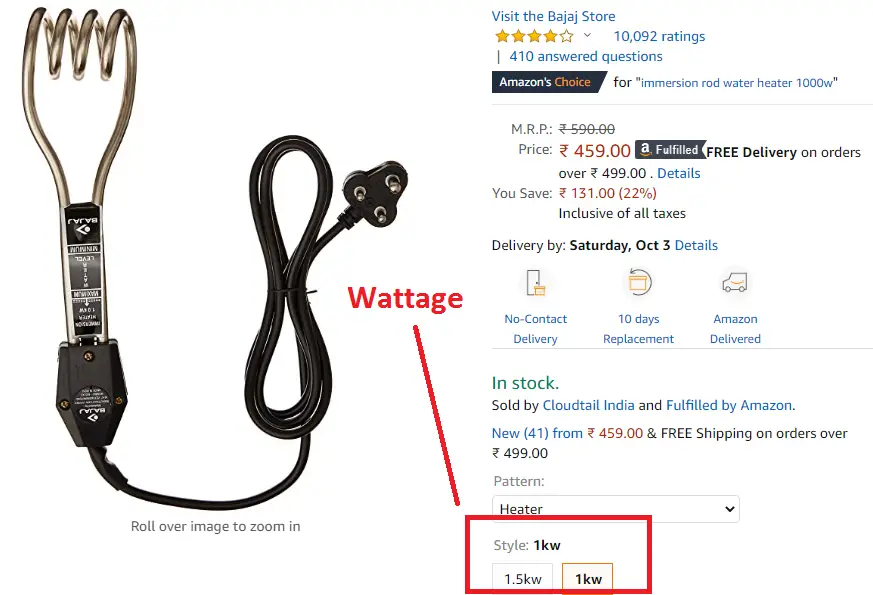# Water Heater Power Consumption Calculation, Power Saving Tips

### Water heater Power Consumption Calculator:

Enter the heater wattage, operating minutes per day and per unit rate. Press the calculate button to get Water Heater Power Consumption per day, per month and per year along with electricity bills.

By default, we have added Mpire Immersion Rod Water Heater 1.5 kW (Color Assorted) (available @ amazon), the operating minutes of 50, and the tariff rate of 3.00/-.

 Enter Minutes Used Per Day: Min Enter Water heater Wattage: W Per Unit rate: Energy Per Day: kWh Energy Per Month: kWh Energy Per Year: kWh Cost Per Hour: Cost Per Day: Cost Per Month: Cost Per Year:

### Water Heater Power Consumption:

The water heater is another useful household which is used for heating the water while bathing, cleaning, drinking etc.

You can see, it looks like a coil or U shape as shown in the figure. Mostly the size of the water heater is measured in wattage and it is available from 500 watts to 2500 watts.

If you do not know, how to calculate the size of the water heater, then use that link.

Note: Here we are talking about water immersion type heater, not the geyser.

Here wattage indicates that the one-hour power consumption will be 500 watts to 2500 watts. However, it contributes 5 to 10% of your electricity bill.

Also, power consumption is depending on how long the water heater is used per day.

Hence, the water heater power consumption is equal to the product of heater on time in hours per day and the heater wattage P(W) in watts divided by 1000. Heater wattage is available @ the nameplate details. The water heater energy consumption formula can be written as,

E(kWh-Heater) = Heater Watts x Operating Hours Per day / 1000

Or

E(kWh-Heater) = P(W) x Hr / 1000

In such a way, per month power consumption is equal to the 30 times of the per day consumption, therefore the formula will be

Learn More:   Cable Diameter Calculation Formula & Excel Calculator For Cable Diameter

E(kWh-Heater) = 30 x Heater Watts x Operating Hours Per day / 1000

For calculating per year consumption, the formula will be,

E(kWh-Heater) = 365 x Heater Watts x Operating Hours Per day / 1000

In some house, you may intend to operate the heater in minutes’ basis means, the consumption will be

E(kWh-Heater) = P(W) x Minutes per day / 60000

Per Month,

E(kWh-Heater) = 30 x P(W) x Minutes per day / 60000

Per year,

E(kWh-Heater) = 365 x P(W) x Minutes per day / 60000

Note: Sometimes, you see water heater wattage will be mentioned in kW, for that, you have multiple the wattage by 1000.For example, look at the image of Bajaj Immersion Rod Water Heater, as per technical specification it consumes 1kW power.

1kW heater wattage = 1 x 1000 = 1000 watts

Look at the table,

 S No kW Watts 1 0.5 500 2 1 1000 3 1.5 1500 4 2 2000 5 2.5 2500 6 3 3000

### Water Heater Power Consumption Calculation:

Let us take one heater for example and we calculate the electricity consumption per day, per month and per year.

Learn More:   LED, LCD, CRT TV & Plasma TV Power Consumption, Wattage

Example:

Let us consider Bajaj 1K watts Immersion Rod Water Heater (available @ amazon) and it operates 45 minutes per day. calculate the energy consumption per day, per month and per year.

Look at the above table it consumes 1000 watts per hour (1 kW per hour).

 Water Heater Power Consumption – 45 minutes Consumption Total (Wh) kWh Hourly 1000 1 Day 750 0.75 Month 22500 22.5 Yearly 270000 270

The water heater consumes 270 units for one year while operating it in 45 minutes per day.

Note that the electricity consumption per day is lesser than the per hour consumption, it means, we are operating it for only 45 minutes.

### Power Savings Tips:

• The main reason for power consumption is, mostly you forget to switch off the heater after reaching the point. So make a timer or alarm to switch off the heater.
• Buy a solar water heater, by the way, you can totally eliminate the electricity consumption of the water heater.
• Try to avoid heating the water under cold condition.